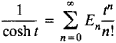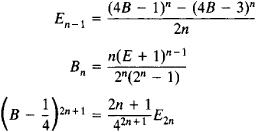# Euler Numbers

Also found in: Wikipedia.
The following article is from The Great Soviet Encyclopedia (1979). It might be outdated or ideologically biased.

## Euler Numbers

in mathematics, the integers En that are the coefficients of tn/n! in the expansion of the function 1/cosh t in the power seriesThese numbers, which were introduced by L. Euler in 1755, are related by the formula (E + 1)n + (E – 1)n = 0, n = 1, 2, 3, . . ., E0 = 1 (after raising to the power, Ek must be inserted for Ek). The following relations hold between Euler numbers and Bernoulli numbers:Euler numbers are encountered in various formulas of mathematical analysis.